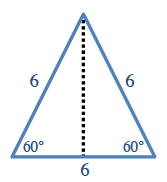### Home > GC > Chapter 11 > Lesson 11.1.5 > Problem11-65

11-65.

Review what you know about polyhedra as you answer the questions below. Refer to the table in problem 11-4 if you need help.

1. Find the total surface area of a regular icosahedron if the area of each face is $45\text{ mm}^2$. Explain your method.

How many sides does an icosahedron have?
Use this to find the surface area.

$900\text{ mm}^2$

2. The total surface area of a regular dodecahedron is $108\text{ cm}^2$. What is the area of each face?

Use the same method you used for part (a).

3. A regular tetrahedron has an edge length of $6$ inches. What is its total surface area? Show all work.

Find the area of one of the faces, and use that to find the total surface area.